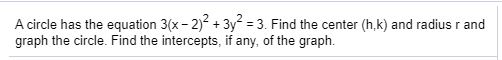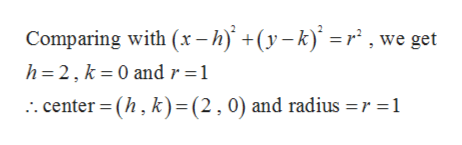# A circle has the equation 3(x-2) +3y 3. Find the center (h,k) and radius r andgraph the circle. Find the intercepts, if any, of the graph.

Question
3 viewshelp_outlineImage TranscriptioncloseA circle has the equation 3(x-2) +3y 3. Find the center (h,k) and radius r and graph the circle. Find the intercepts, if any, of the graph. fullscreen
check_circle

Step 1

Given,

Step 2

On simplification, we get

Step 3

Now comparing with standard equ...help_outlineImage TranscriptioncloseComparing with (x-h) +(y-k)= r , we get h 2, k 0 and r =1 center (h, k)= (2,0) and radius =r =1 fullscreen

### Want to see the full answer?

See Solution

#### Want to see this answer and more?

Solutions are written by subject experts who are available 24/7. Questions are typically answered within 1 hour.*

See Solution
*Response times may vary by subject and question.
Tagged in

### Other